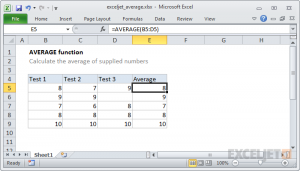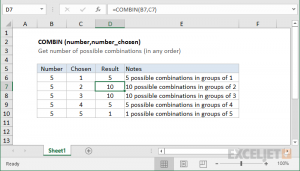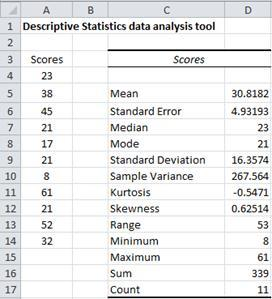# Microsoft Excel Standard Error – What Is It And How To Measure It In 2 Different Methods

The standard error or, as it is often called, the arithmetic mean error, is one of the important statistical indicators. Using this indicator, you can determine the heterogeneity of the sample. It is also quite important in forecasting. Let’s find out in what ways you can calculate the standard error value using Microsoft Excel tools.

## Calculation of arithmetic average errorOne of the indicators that characterize the integrity and homogeneity of the sample is the standard error. This value is the square root of the variance.

The variance itself is the square mean of the arithmetic mean. The arithmetic average is calculated by dividing the total value of the sample objects by their total number.

In Excel, there are two ways to calculate the standard error: using a set of functions and using the tools of the Analysis Package. Let’s take a closer look at each of these options.

### Method 1: Calculate using a combination of functionsFirst of all, let’s create an algorithm of actions on a specific example for calculating the arithmetic mean error, using a combination of functions for these purposes. To perform the task, we need the operators STANDOCLON.VROOT and ACCOUNT.

For example, we will use a sample of twelve numbers presented in the table.

1. Select the cell in which the total value of the standard error excel will be displayed, and click on the “Insert Function” icon.
2. The function wizard opens. We make a move to the block “Statistical.” In the presented list of names, choose the name “STANDOCLON.V.”
3. The argument window of the above statement is launched. STDEV.V is designed to estimate the standard deviation of the sample. This statement has the following syntax:

= STANDEVRECH.V (number1; number2; …)

“Number1” and the following arguments are numeric values ​​or references to cells and sheet ranges in which they are located. There can be up to 255 arguments of this type. Only the first argument is required.

So, set the cursor in the field “Number1”. Next, make sure to clamp the left mouse button, select the entire range of the sample on the sheet with the cursor. The coordinates of this array are immediately displayed in the field of the window. After that, we click on the “OK” button.

1. In the cell on the sheet displays the result of the calculation of the operator STANDEVKLON.V. But this is not an arithmetic mean error. In order to obtain the desired value, the standard deviation should be divided by the square root of the number of sample elements. In order to continue the calculations, select the cell containing the function STANDEPCLON.V. After this, we place the cursor in the formula line, and after the already existing expression we add the division sign ( / ). Following this, we click on the icon of a triangle turned upside down, which is located to the left of the formula bar. A list of recently used functions opens. If you find in it the name of the operator “ROOT,” then go on this name. Otherwise, click on the item “Other functions …”.
2. The function wizard starts up again. This time we should visit the “Mathematical” category. In the presented list, select the name “ROOT” and click on the “OK” button.
3. The arguments window of the ROOT function opens. The only task of this operator is to calculate the square root of a given number. Its syntax is extremely simple:

= ROOT (number)

As you can see, the function has only one argument “Number.” It can be represented by a numeric value, a reference to the cell in which it is contained, or another function that calculates this number. The last option will be presented in our example.

We set the cursor in the “Number” field and click on the familiar triangle that brings up the list of recently used functions. We look for in it the name “ACCOUNT.” If we find, then click on it. In the opposite case, again, go by the name “Other Functions …”.

1. In the opened window of the Function Wizard, we move to the “Statistical” group. There we select the name “ACCOUNT” and perform a click on the “OK” button.
2. The arguments window of the ACCOUNT function is launched. The specified operator is designed to calculate the number of cells that are filled with numeric values. In our case, it will count the number of sample elements and report the result to the “parent” operator ROOT. The function syntax is as follows:

= ACCOUNT (value1; value2; …)

The “Value” arguments, which can be up to 255 pieces, are references to cell ranges. Put the cursor in the field “Value1”, hold down the left mouse button and select the entire range of the sample. After its coordinates are displayed in the field, click on the “OK” button.

1. After the last action, the number of cells filled with numbers will not only be calculated, but the arithmetic average error will also be calculated since this was the final stroke in the work on this formula. The magnitude of the standard error is derived in the cell where the complex formula is located, the general form of which in our case is the following:

= STDEV.V (B2: B13) / ROOT (ACCOUNT (B2: B13))

The result of the calculation of the average arithmetic error was 0.505793. Let’s remember this number and compare it with the one we get when solving the problem in the following way.

But the fact is that for small samples (up to 30 units) for better accuracy it is better to use a slightly modified formula. In it, the standard deviation value is divided not by the square root of the number of sample elements, but by the square root of the number of sample elements minus one. Thus, taking into account the nuances of a small sample, our formula will take the following form:

= STDEV.V (B2: B13) / ROOT (ACCOUNT (B2: B13) -1)

### Method 2: Use the Descriptive Statistics ToolThe second embodiment, by which one can calculate the standard error in Excel, is the use of a tool “Descriptive Statistics,” included in the toolkit “Data Analysis” ( “Analysis Package” ). “Descriptive Statistics” conducts a comprehensive analysis of the sample according to various criteria. One of them is just finding the arithmetic mean error.

But to take advantage of this opportunity, you need to immediately activate the “Analysis Package,” since it is disabled by default in Excel.

1. After the document is opened with a selection, go to the “File” tab.
2. Next, using the left vertical menu, move through its item in the “Parameters” section.
3. The Excel parameters window starts. In the left part of this window, there is a menu through which we move to the “Superstructures” subsection.
4. At the very bottom of the window that appears, there is a “Management” field. We set in it the parameter “Excel add-ins” and click on the button “Go …” to its right.
5. The add-ons window starts with a list of available scripts. Check the name “Analysis Package” and click on the “OK” button in the right part of the window.
6. After the last action, a new group of tools will appear on the ribbon, which has the name “Analysis.” To go to it, click on the name of the tab “Data.”
7. After the transition, click on the button “Data Analysis” in the block of tools “Analysis,” which is located at the very end of the tape.
8. The analysis tool selection window starts. Select the name “Descriptive Statistics” and click on the “OK” button on the right.
9. The settings window of the Descriptive Statistics complex statistical analysis tool is launched.

In the field “Input interval,” you must specify the range of table cells in which the analyzed sample is located. It is inconvenient to do this manually, although it is possible, so we put the cursor in the specified field and, with the left mouse button held down, select the corresponding data array on the sheet. His coordinates will be immediately displayed in the field of the window.

In the “Grouping” block, we leave the default settings. That is, the switch should stand near the item “By columns.” If not, then it should be rearranged.

The tick “Tags in the first line” can not be set. For the solution to our question is not important.

Next, go to the settings “Output Parameters.” Here you should specify exactly where the result of the “Descriptive Statistics” tool will be displayed :

• On a new sheet;
• In a new book (another file);
• In the specified range of the current sheet.

Let’s select the last of these options. To do this, move the switch to the “Output interval” position and set the cursor in the field opposite this parameter. After that, we click on the sheet by the cell, which will become the top left element of the data output array. Its coordinates should be displayed in the field in which we previously set the cursor.

The following is a settings block that determines which data you need to enter:

• Summary statistics;
• Q the largest;
• The smallest;
• Level of reliability

To determine the standard error, it is necessary to tick the “Total statistics” option. Opposite the rest of the items, we tick at our discretion. It will not affect the solution of our main task in any way.

After all the settings in the “Descriptive statistics” window are set, click on the “OK” button in its right side.

1. After this, the Descriptive Statistics tool displays the results of sample processing on the current sheet. As you can see, there are quite a lot of diverse statistical indicators, but among them, there is the one we need – “Standard Error.” It is equal to the number 0,505793. This is exactly the same result that we achieved by applying a complex formula when describing the previous method.

As you can see, in Excel, you can calculate the standard error in two ways: using the set of functions and using the Descriptive Statistics analysis tool. The final result will be exactly the same.

Therefore, the choice of method depends on the convenience of the user and the specific task. For example, if the arithmetic means the error is only one of many statistical sampling indicators that need to be calculated, it is more convenient to use the Descriptive Statistics tool.

But if you only need to calculate this indicator, then in order to avoid cluttering up extra data, it is better to resort to a complex formula. In this case, the result of the calculation fits in one cell of the sheet.